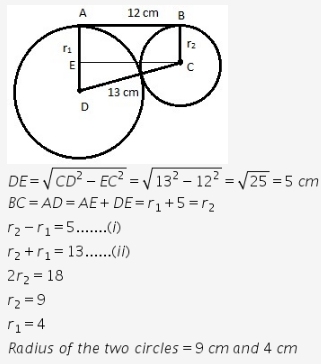# If the length of a direct common tangent to two circles be 12cm and the length

If the length of a direct common tangent to two circles be 12cm and the length of a Transverse common tangent to them be 5cm.Find the Radii of two circles,given that the distance between their cetres is 13cm

1 Like1 Like